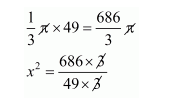Question:

A hemisphere of lead of radius 7 cm is cast into a right circular cone of height 49 cm. Find the radius of the base.

Solution:

Radius of hemisphere r = 7 cm

The volume of hemisphere

$=\frac{2}{3} \pi r^{3}$

$=\frac{2}{3} \pi \times(7)^{3}$

$=\frac{2}{3} \pi \times 343$

$=\frac{686}{3} \pi \mathrm{cm}^{3}$

Since, the hemisphere cast into the right circular cone

The height of cone h = 49 cm

Let x be the radius of cone.

Clearly,

Volume of cone = volume of hemisphere$=14$

$x^{2}=14$

$x=\sqrt{14}$

$x=3.74 \mathrm{~cm}$

Thus, the radius of cone = 3.74 cm